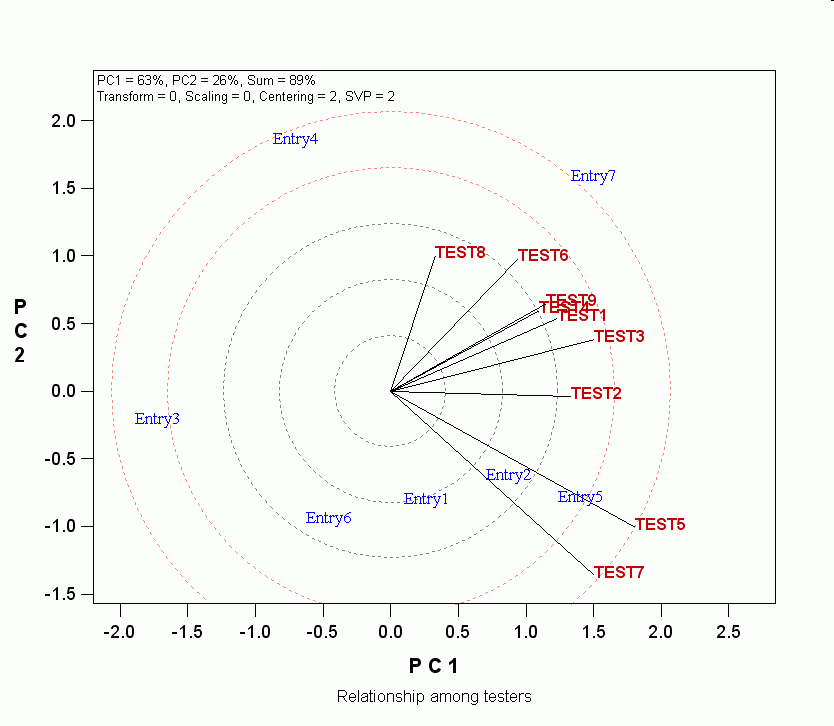Contents - Index

Show relationship among testers

From Biplot Analysis, click Relation among Testers, and the vectors of the testers will show on the biplot. If the biplot was not in the tester-focused singular value partitioning (i.e., SVP = 2), this method will be automatically applied, which may change the biplot shape.Interpretation:

• When the data is sufficiently approximated by the biplot (How do I know it is sufficientlinkID=35}), the cosine of the angle between the vectors of two testers approximates the correlation coefficients between them.
• Two testers are positively correlated if the angle between their vectors is <90°;
• Two testers are negatively correlated if the angle between their vectors is >90°;
• Two testers are independent if the angle between them is 90°.
• 0° means r =1.
• 180° means r = -1.
• Testers with longer vectors are more discriminating of the entries; those with short vectors are less discriminating; those located at the biplot origin are not discriminating.
• Caution: These interpretations are valid only for GGE biplots that are not sacled by within tesster standard-deviations)

Applications

• Closely associated testers may suggest redundant testers (e.g., test environments)
• Closely associated testers may be used in identifying variables (traits or makers) that can be used in indirect selection for a target variable.
• Large gaps between testers may suggest that additional testers are needed to fully sample the target population. Alternatively, it may indicate that the testers fall in groups.
• The largest angle in a biplot may be used as an indicator of the size of entry by tester interaction. The more the number of negative correlations, the larger the interaction between the entries and the testers.

Note

• When the biplot explain only a small portion of the total variation, say, <50%, the angles may not exactly reflect the correlations among the testers.
• The accuracy of the relationships among testers as shown in the biplot can be examined by requesting a correlation matrix among testers.